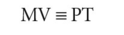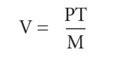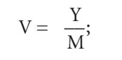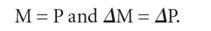# quantity theory of money

Also found in: Dictionary, Wikipedia.
Related to quantity theory of money: Velocity of money

## Quantity Theory of Money

The idea that the amount of money in an economy directly correlates to the price of goods and services. According to this theory, more money in an economy results in higher prices. Nearly all economists agree with the quantity theory of money in the long run, but there is significant disagreement over how true it is in the short term. There is also disagreement over what kinds of money (cash, notes or others) affect prices. The quantity theory of money is a major component of monetarism. See also: Real bills doctrine.
Farlex Financial Dictionary. © 2012 Farlex, Inc. All Rights Reserved

## quantity theory of money

see MONETARISM.
Collins Dictionary of Business, 3rd ed. © 2002, 2005 C Pass, B Lowes, A Pendleton, L Chadwick, D O’Reilly and M Afferson

## quantity theory of money

a theory that posits a direct relationship between the MONEY SUPPLY and the general PRICE LEVEL in an economy.

The basic identity underlying the quantity theory was first developed by Irving Fisher (1867–1947) in 1911. The Fisher equation states that:where M is the money stock, V is the VELOCITY OF CIRCULATION of money (the average number of times each £ or \$ changes hands in financing transactions during a year), P is the general price level, and T is the number of transactions or the total amount of goods and services supplied.

The above relationship is true by definition because total money expenditure on goods and services (MV) in a period must equal the money value of goods and services produced (PT), and the four terms are defined in such a way that the identity must hold. However, the identity can be converted into a testable equation by assuming that the velocity of circulation of money is constant or changes slowly.

Economists at Cambridge University reformulated the traditional quantity theory of money to emphasize the relationship between the stock of money in an economy (M) and final income (Y), of the form MV = Y. The income velocity of circulation (Cambridge equation) is thus:where V is the average number of times the money stock of an economy changes hands in the purchase of final goods and services. For example, taking Y as gross national product, if a country has a GNP of £5,000 million and an average money stock (M) over a year of £1,000 million, then V is 5. Velocity cannot be observed directly and is thus determined using Y and M, figures that may be calculated from government statistics.

The term V in the Cambridge equation is not the same as V in Fisher's traditional quantity theory of money In Fisher's equation, MV = PT, rearranged to give:the number of transactions in the period, T, includes all transactions for real goods and services plus financial transactions. In the Cambridge equation, PT (where P = average price level) is replaced by Y, which contains not all transactions but only those generating final income. This formulation allowed the Cambridge economists to emphasize real income (that is, final goods and services).

The classical economists argue that velocity of circulation was constant because consumers have relatively constant spending habits and so turn over money at a steady rate. This argument converts the identity into an equation that leads to the quantity theory that expresses a relationship between the supply of money and the general price level. If V and T are constant, then:The modern exponents of the quantity theory (see MONETARISM) do not necessarily hold that the velocity of circulation is fixed, but they argue that it will change only slowly over time as a result of financial innovations, like the spread of bank accounts and cheque payments and the growing use of credit cards. They also point out that in a fully employed economy there is a maximum amount of goods and services being produced and which therefore can be exchanged, so the number of transactions, T, is determined by real supply-wide considerations, like productivity trends. With V and T fixed or slowly changing, then the price level is determined by the stock of money, M. Any increase in the money supply feeds directly into an increase in demand for goods and services (AGGREGATE DEMAND). It follows that if the money supply (M), and hence aggregate demand, increase over time faster than the supply capacity of the economy (T), the result will be a rise in the general price level, P (INFLATION). By contrast, Keynesian economists argue that the velocity of circulation is unstable and changes rapidly and may offset changes in the money stock. See alsoTRANSMISSION MECHANISM, MONEY SUPPLY/SPENDING LINKAGES, MONETARY POLICY.

Collins Dictionary of Economics, 4th ed. © C. Pass, B. Lowes, L. Davies 2005
References in periodicals archive ?
(1974), The Quantity Theory of Money: Its Historical Evolution and Role in Policy Debate, Economic Review.
Following the quantity theory of money, central banks accumulated foreign currency reserves in order to sterilise excess liquidity caused by foreign currency imports.
Correspondingly, it turns out that the lack of inflationary pressure reflects a collapse not in so-called 'velocity' in the quantity theory of money, but instead in the so-called 'money multiplier' relating the effective money supply in the economy to the money base of currency plus bank reserves at the Federal Reserve.
As a result, even if the Bank of Japan (BOJ) increases the monetary base, the quantity theory of money does not apply, and the price level does not rise by much.
Rather than a quantity theory of money, we need a disaggregated quantity theory of credit.
Accepting some form of the quantity theory of money, then, we have a theory of inflation.
And finally, the fourth Misesian insight I will discuss is the nature of the liquidity effect, and how it relates the so-called "Taylor Rule" to the Quantity Theory of Money.
The first theory on money is quantity theory of money that is presented by Fischer.
At the forefront of this challenge was Milton Friedman, who reformulated and improved the quantity theory of money. He won the 1976 Nobel Prize in economics for his work in monetary economics and related advances in economic theory.
"The Quantity Theory of Money: A Restatement," in Milton Friedman, ed., Studies in the Quantity Theory of Money, Chicago: University of Chicago Press.
"The Quantity Theory of Money." In The New Palgrave Money, edited by John Eatwell, Murray Milgate, and Peter Newman.

Site: Follow: Share:
Open / Close